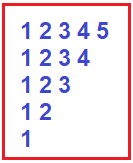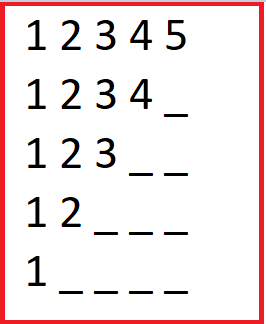# Inverted Pyramid of Numbers Program in C#

## Inverted Pyramid of Numbers Pattern Program in C#

In this article, I am going to discuss How to implement the Inverted Pyramid of Numbers Pattern Program in C# with Examples. Please read our previous article where we discussed Hollow Right Triangle Star Pattern Program in C#. Please have a look at the below image which shows the Inverted Pyramid of Numbers Pattern.##### Understanding Pyramid of Numbers Star Pattern

If we want to create an inverted pyramid of numbers with five rows, then

1. In the first row, we have 1,2,3,4, 5, and 0 blank spaces.
2. In the second row, we have 1,2,3, 4, and 1 blank spaces.
3. In the third row, we have 1,2, 3, and 2 blank spaces.
4. In the fourth row, we have 1 and 2, and 3 blank spaces.
5. In the fifth row, we have 1 and 4 blank spaces as shown in the below image.1. From the above pattern, it is clear that in each row numbers are decreasing by -1 at each row.
2. For example, the 1st row has 1,2,3,4 and 5, the 2nd row has 1,2,3 and 4 and the 3rd row has 1,2 and 3, and so on.
3. From the above pattern, it is clear that at each row number of blank spaces is increasing +1 at each row. For example, the 1st row has 0 spaces, the 2nd row has 1 space, the 3rd row has 2 spaces, and so on.
##### Calculate the number to be printed in each row

Suppose if the number of rows is 5 then

1. In the first row, we have numbers up to 5(equal to row).
2. In the second row, we have numbers up to 4 (row-1).
3. In the third row, we have numbers up to 3 (row-2).
4. In the fourth row, we have numbers up to 2 (row -3).
5. In the fifth row, we have 1 (row – 4).
##### How to Implement Inverted Pyramid of Numbers Program in C#?

To solve the above pattern,

1. We are going to use two for loops. One outer for loop and one inner for loop.
2. The outer for loop will be used to handle the rows one by one and the two inner for loops will be used to handle the columns one by one.
3. The inner loop will be used to print the numbers according to row and the outer loop’s job is to go on a new line after printing the current row numbers.
##### Program to Print Inverted Pyramid of Numbers Pattern in C#

The following C# Program will allow the user to input the number of rows and then print an inverted pyramid of numbers on the console

```using System;
namespace PatternDemo
{
public class InvertedPyramidOfNumbersPattern
{
public static void Main()
{
Console.Write("Enter Number of Rows :");
for (int i = rows; i >= 1; i--)
{
//j<=i  //let rows = 5
/* first row (0 to 5) = 5 numbers (1,2,3,4,5)
* second row (0 to 4) = 4 numbers (1,2,3,4)
* third row (0 to 3) = 3 numbers (1,2,3)
* and so on
*/
//calculate stars for each row one by one
for (int j = 1; j <= i; j++)
{
Console.Write(j + " ");
}
Console.WriteLine();
}